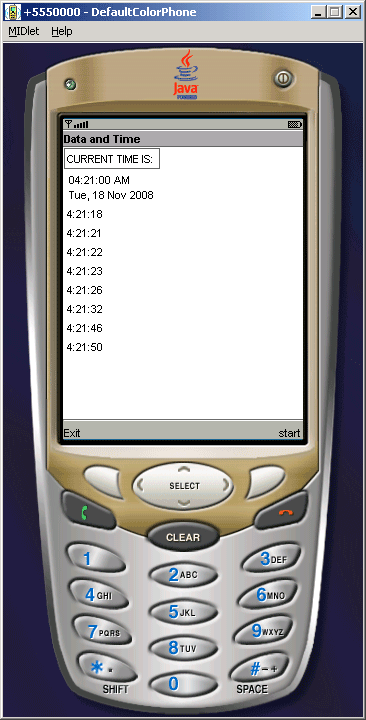# J2ME Current Date And Time

This is a simple J2ME form example, that is going to show the current date and time on the screen.# J2ME Current Date And Time

This is a simple J2ME form example, that is going to show the current date and time on the screen. Like core Java J2ME too use the same  java.util package to show the current date as well as current time on the screen.  In this Midlet we have set the current date and time as date & time. That's why it is going to show you current date and time, every time you runs the application.

The output of the application will look like as below given image..First of all in this image you can see that we have created a form called Date & Time and set the current time and date as Item.

Source Code of DateAndTime.java

 ``` import java.util.*; import javax.microedition.lcdui.*; import javax.microedition.midlet.*; public class DateAndTime extends MIDlet implements CommandListener{   private Display disp;   private Date d;   Calendar c = Calendar.getInstance();   String time;   private DateField currentDate;   private Command start, exit;   private Form form;   private int index;   public DateAndTime(){   form = new Form("Data and Time");   d = new Date();   start = new Command("start", Command.SCREEN, 1);   exit = new Command("Exit", Command.EXIT, 0);   currentDate = new DateField("", DateField.DATE_TIME);   currentDate.setDate(d);   }      public void startApp(){   form.append("CURRENT TIME IS: ");   index = form.append(currentDate);   form.addCommand(start);   form.addCommand(exit);   form.setCommandListener(this);   disp = Display.getDisplay(this);   disp.setCurrent(form);   }   public void pauseApp(){}   public void destroyApp(boolean uncond){}   public void commandAction(Command cmd, Displayable s){   if (cmd == exit){   notifyDestroyed();   }else if (cmd == start){   d = new Date();   c.setTime(d);   time = c.get(Calendar.HOUR_OF_DAY) + ":" +c.get(Calendar.MINUTE)     + ":" + c.get(Calendar.SECOND);   form.append("" + time);   form.append("\n");   }   } }```